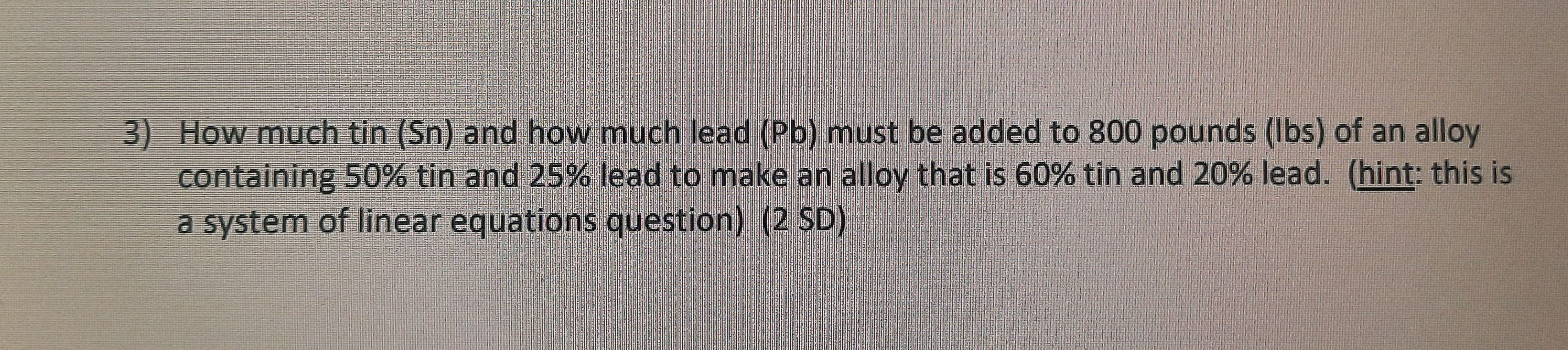### Still have math questions?

Algebra
QuestionHow much tin (Sn) and how much lead (Pb) must be added to $$800$$ pounds (Ibs) of an alloy containing $$50 \%$$ tin and $$25 \%$$ lead to make an alloy that is $$60 \%$$ tin and $$20 \%$$ lead. (hint: this is

a system of linear equations question) (2 SD)

$$200pounds\ Sn\\0pounds\ Pb$$